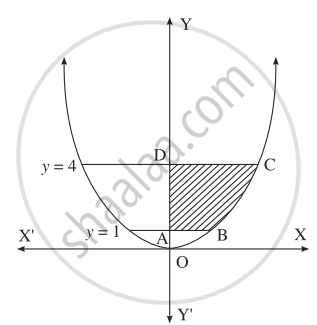# Solve the following : Find the area of the region bounded by the curve y = 4x2, Y-axis and the lines y = 1, y = 4. - Mathematics and Statistics

Sum

Solve the following :

Find the area of the region bounded by the curve y = 4x2, Y-axis and the lines y = 1, y = 4.

#### SolutionBy symmetry of the parabola, the required area is 2 times the area of the region ABCD.

From the equation of the parabola, x2 = y/(4)

the first quadrant, x > 0

∴ x = (1)/(2)sqrt(y)

∴ required area = int_1^4 x*dy

= (1)/(2) int_1^4 sqrt(y)*dy

= (1)/(2)[y^(3/2)/(3/2)]_1^4

= (1)/(2) xx (2)/(3)[4^(3/2) - 1^(3/2)]

= (1)/(3)[(2^2)^(3/2) - 1]

= (1)/(3)[8 - 1]

= (7)/(3)"sq units".

Concept: Area Bounded by the Curve, Axis and Line
Is there an error in this question or solution?

#### APPEARS IN

Balbharati Mathematics and Statistics 2 (Arts and Science) 12th Standard HSC Maharashtra State Board
Chapter 5 Application of Definite Integration
Miscellaneous Exercise 5 | Q 2.1 | Page 190